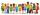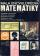# Stairs

Between adjacent levels are 15 stairs. If step will be 1.2 cm lower there would be 16 stairs. What is the stair height?

Result

x =  19.2 cm

#### Solution:

15x = 16(x-1.2)

x = 19.2

x = 965 = 19.2

Calculated by our simple equation calculator.

Leave us a comment of example and its solution (i.e. if it is still somewhat unclear...):

Showing 0 comments:Be the first to comment!#### To solve this example are needed these knowledge from mathematics:

Do you have a linear equation or system of equations and looking for its solution? Or do you have quadratic equation? Do you want to convert length units?

## Next similar examples:

1. The classroomIn the seventh class the number of boys and girls are at a ratio of 4:5. Boys are 16. How many total students are in the classroom?
2. One-thirdA one-third of unknown number is equal to five times as great as the difference of the same unknown number and number 28. Determine the unknown number.
3. BookTo number pages of thick book was used 4201 digits. How many pages has this book?
4. Unknown number 11That number increased by three equals three times itself?
5. QuizTested student answered correctly on a 4/ 8 questions. Wrong answers was 16. How many questions answered?
6. FactoryIn the factory workers work in three shifts. In the first inning operates half of all employees in the second inning and a third in the third inning 200 employees. How many employees work at the factory?
7. Forest nurseryIn the forest nursery after winter, they found that 1/10 stems died out of them. For them, they land 193 new spruces. How many spruces are in the forest nursery?
8. Equation?
9. Negative in equation2x + 3 + 7x = – 24, what is the value of x?
10. CakesGrandmother baked cakes. Half of its was poppy, quarter with plum jam and 16 cheesecakes. How many cakes she baked in total?
11. Unknown numberIdentify unknown number which 1/5 is 40 greater than one tenth of that number.
12. 1.5 divided1.5 divided by 1 = w divided by 4
13. Dropped sheetsThree consecutive sheets dropped from the book. The sum of the numbers on the pages of the dropped sheets is 273. What number has the last page of the dropped sheets?
14. Simple equationSolve the following simple equation: 2. (4x + 3) = 2-5. (1-x)
15. Find xSolve: if 2(x-1)=14, then x= (solve an equation with one unknown)
16. InfirmaryTwo thirds of children from the infirmary went on a trip seventh went to bathe and 40 children remained in the gym. How many children were treated in the infirmary?
17. Equation 29Solve next equation: 2 ( 2x + 3 ) = 8 ( 1 - x) -5 ( x -2 )